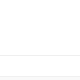## Welcome to the Treehouse Community

Want to collaborate on code errors? Have bugs you need feedback on? Looking for an extra set of eyes on your latest project? Get support with fellow developers, designers, and programmers of all backgrounds and skill levels here with the Treehouse Community!

### Looking to learn something new?

Treehouse offers a seven day free trial for new students. Get access to thousands of hours of content and join thousands of Treehouse students and alumni in the community today.# So, what's up with my code, it wont print the last line.

```var qa = [
['what is 1+1', 2],
['what is 1+2', 3],
['what is 1+3', 4]
];
var correctA = 0;
var q;
var a;

function print(message) {
document.write(message);
}

for ( var i = 0; i <= qa.length; i +=1) {
q = parseInt(prompt(qa[i]));
a = qa[i];
if ( a === q ){
correctA +=1;
}else {
correctA -=1;
}

}

print('<h2>Correct answers: ' + correctA + '</h2>');
```You need to change your `for` loop statement to run the same amount of times as `qa.length`.
Currently you have `i = 0` and `i <= qa.length`. Since `qa.length` is equal to 3, the loop is attempting to run 4 times.
1. Set `i` to 1
2. Have `i < qa.length` (i.e remove the equals sign, from "<=" to be "<")